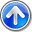# The ZhemweL

Seputar Dunia IT dan Fakta Unik Lainnya

## Thursday, 15 June 2017

MATLAB is a high-level technical computing language and interactive environment for algorithm development, data visualization, data analysis, and numeric computation. Using the MATLAB product, you can solve technical computing problems faster than with traditional programming languages, such as C, C++, and Fortran.

MATLAB provides a number of features for documenting and sharing your work. You can integrate your MATLAB code with other languages and applications, and distribute your MATLAB algorithms and applications.

You can use MATLAB in a wide range of applications, including signal and image processing, communications, control design, test and measurement, financial modeling and analysis, and computational biology. Add-on toolboxes (collections of special-purpose MATLAB functions, available separately) extend the MATLAB environment to solve particular classes of problems in these application areas.

### Key Features

• Mathematical functions for linear algebra, statistics, Fourier analysis, filtering, optimization, and numerical integration
• 2-D and 3-D graphics functions for visualizing data
• Tools for building custom graphical user interfaces
• Functions for integrating MATLAB based algorithms with external applications and languages, such as C, C++, Fortran, Java, COM, and Microsoft Excel
• High-level language for technical computing
• Development environment for managing code, files, and data
• Interactive tools for iterative exploration, design, and problem solving

1.2.terimakasih gan

3.thankss gann

4.terimakasih

5.broken bro

BILA ANDA MEMASUKKAN LINK HIDUP, MAKA AKAN OTOMATIS TERDELETE..